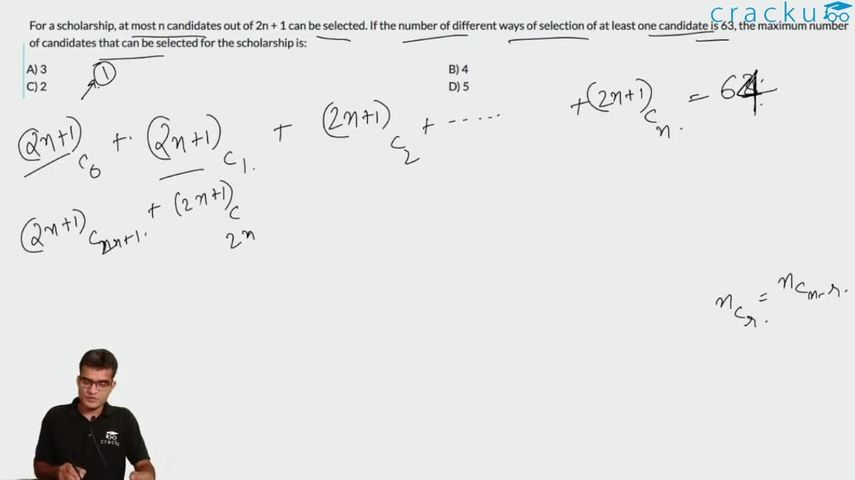Question 5

# For a scholarship, at most n candidates out of 2n + 1 can be selected. If the number of different ways of selection of at least one candidate is 63, the maximum number of candidates that can be selected for the scholarship is:

Solution

At least one candidate and at most n candidates among 2n+1 candidates =>

^{2n+1}C_1Total number of ways alteast one candidate can be selected = Total number of ways of selecting a candidate - total number of ways of selecting no candidates

$$\Rightarrow ^{2n+1}C_1+^{2n+1}C_2+^{2n+2}C_3+....+^{2n+1}C_{n-1}+^{2n+1}C_n = 63$$

We know that $$^{2n+1} C_0$$ and $$^{2n+1} C_{2n+1}$$ are equal to 1.

By Binomial Expansion

$$^{2n+1}C_0+^{2n+1}C_1$$+... + $$^{2n+1}C_{2n}+^{2n+1}C_{2n+1} = (1+1)^{2n+1}$$ ---- Eq 1

Also $$^{2n+1}C_1$$ = $$^{2n+1}C_{2n}$$ by symmetry

and $$^{2n+1}C_2$$ = $$^{2n+1}C_{2n-1}$$ and so on

So $$\Rightarrow ^{2n+1}C_{n+1}+^{2n+1}C_{n+2}+....+^{2n+1}C_{2n-1}+^{2n+1}C_{2n} = 63$$

Therefore, on substituting these values in Eq 1 we get

1 + 63 + 63 +1 = $$2^{2n+1}$$

$$2^{2n+1}$$ = 128

2n+1 = 7

Therefore, n=3

As at most n students can be selected, the correct answer is 3.

### View Video Solution